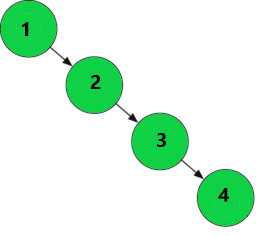# Balanced Binary Tree – Definition, How to Check, Time & Space Complexity

In this article, we take a look into an important type of Binary Tree based Data Structure – Balanced Binary Tree. We will discuss the description of balanced binary trees with examples. Along with this, we will also look at an interesting problem related to it.

## Definition

A Balanced Binary Tree commonly referred to as Height-Balanced Binary Tree, is a binary tree in which the depth of the two subtrees on either side of every node never differs by more than 1. For any node, the difference in height for its left and right subtrees respectively does not exceed 1. The height difference can have a value either 0 or 1. The tree is said to be balanced if the height of the tree is maintained at Log n at every instant, for n nodes in a tree. Similarly, a Binary Search Tree that follows the above condition is a Balanced Binary Search Tree or Balanced BST. So, to summarize in Balanced BST:

• The difference in height of left and right subtrees must not exceed 1.
• And, the left subtree and the right subtrees must be balanced.

Let us consider this example:Note: For every node, HD -> Height Difference = Height of Left Subtree – Height of Right Subtree. For Leaf Nodes HD is always 0 because they have no children.

In the above example, we see a Balanced binary tree. Each node follows the given condition discussed above for each of its subtree. Node 2 has HD value 0 because height of its left subtree is 1 and height of right subtree is also 1, their difference is 0. In similar way, we calculate HD values for every node. The above is also an example of Balanced Binary Search Tree.

## Why Use Balanced Binary Tree?

Binary Trees or Binary Search Tree are useful data structures when it comes to store data in a Hierarchical format or in an Ordered manner. They also try to optimize the lookup time in our tree to O (log n), but this is not the case when the given binary tree is skewed in nature or for Skewed Binary Search Trees.

Consider this tree for example:This is a Right Skewed Binary Search Tree, where all the nodes are present in only the right side of the root and its children, keeping binary search tree property intact. This is not a branching tree, but a linear tree. All of the left subtrees are empty. Because of this, the worst case occurs for the search operation and other operations as well (insertion and deletion) taking O(n) time. From this perspective, it is viable to use a linked list and do linear search. So, for this reason, we use Balanced Binary Trees to optimize the time for all the operations- Search, Insert, Delete always remain O(log n). After each operation, the tree must remain Height-Balanced to avoid the worst case.

## How to Check?

Now let us have a look at the problem ‘Given a Binary Tree, Determine If It is Balanced‘.

Note: In Parentheses, The Height Difference value of every node is shown.

### Approach:

We follow these steps to check whether a given binary tree is balanced or not.

• We first calculate the height for each node starting from the leaf nodes basically performing Post-Order Traversal.
• Then, for each node we calculate the height of its left subtree and the right subtree and check whether the difference of their heights is greater than 1. If at any instant we see the height difference is more than 1, it means our tree is Unbalanced.
• We have a Boolean variable isBalanced which keeps track at what instant the height difference>1. As soon as we find it, we assign it value false indicating that the tree is Unbalanced. If it stays true the entire time it means our tree is Balanced.

### Implementation in Java

Let us look at the implementation of the above approach in Java:

Output:

## Time Complexity

Basically, we are traversing all nodes of the binary tree to determine their height, so the Time Complexity to check whether a tree is balanced is O(n), for n nodes in the tree.

## Space Complexity

We followed a recursive approach to implement the solution which makes at most N number of Recursive Calls. So, if System Space is considered for each call, then Space Complexity is O(n).

That’s all for Balanced Binary Trees, you can try this code with different examples (like Example 2) and run it in your local Java Compiler to get a clear idea of the working.

You can leave your suggestions or doubts in the comment section below.